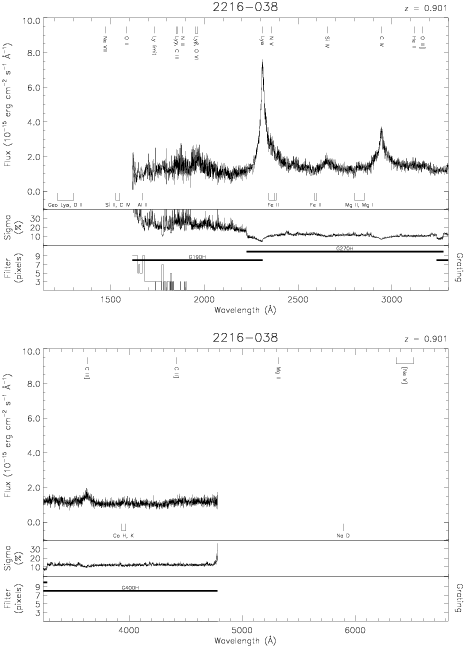Download dataset
TELESCOP= 'HST     '
INSTRUME= 'FOS     '
EQUINOX =               2000.0
DATE-OBS= '1993-10-03T18:44:08'
RA_PNT  =       334.7170000000
DEC_PNT =        -3.5937222222
PA_PNT  =       184.8096008301
OBJECT  = '2216-038'
ROOTNM01= 'Y1EM0102P'
GRNDMD01= 'SPECTROSCOPY'
DETECT01= 'AMBER   '
APERID01= 'A-1     '
FGWAID01= 'H19     '
ROOTNM02= 'Y1EM0103P'
GRNDMD02= 'SPECTROSCOPY'
DETECT02= 'AMBER   '
APERID02= 'A-1     '
FGWAID02= 'H27     '
ROOTNM03= 'Y1EM0104P'
GRNDMD03= 'SPECTROSCOPY'
DETECT03= 'AMBER   '
APERID03= 'A-1     '
FGWAID03= 'H40     '